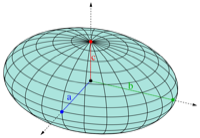Cococubed.com Coating an ellipsoid

Home

Astronomy research
Software instruments
Stellar equation of states
EOS with ionization
EOS for supernovae
Chemical potentials
Stellar atmospheres

Voigt Function
Jeans escape
Polytropic stars
Cold white dwarfs

Cold neutron stars
Stellar opacities
Neutrino energy loss rates
Ephemeris routines
Fermi-Dirac functions

Polyhedra volume
Plane - cube intersection
Coating an ellipsoid

Nuclear reaction networks
Nuclear statistical equilibrium
Laminar deflagrations
CJ detonations
ZND detonations

Fitting to conic sections
Unusual linear algebra
Derivatives on uneven grids

Supernova light curves
Exact Riemann solutions
1D PPM hydrodynamics
Hydrodynamic test cases
Galactic chemical evolution

Universal two-body problem
Circular and elliptical 3 body
The pendulum
Phyllotaxis

MESA
MESA-Web
FLASH

Zingale's software
Brown's dStar
GR1D code
Herwig's NuGRID
Meyer's NetNuc
Presentations
Illustrations
Public Outreach
Education materials
2023 ASU Solar Systems Astronomy
2023 ASU Energy in Everyday Life

AAS Journals
2023 AAS Peer Review Workshops
2023 MESA VI
2023 MESA Marketplace
2023 MESA Classroom
2023 Neutrino Emission from Stars
2023 White Dwarfs & 12C(α,γ)16O
2022 Earendel, A Highly Magnified Star
2022 Black Hole Mass Spectrum
2022 MESA in Don't Look Up
2021 Bill Paxton, Tinsley Prize

Contact: F.X.Timmes
my one page vitae,
full vitae,
research statement, and
teaching statement.

Let's start from something familiar and then generalize it. Consider sphere of radius r. Increase the radius by a distance d. The new volume is \begin{equation} \dfrac{4}{3} \pi (r + d)^3 = \dfrac{4}{3} \pi (r^3 + 3 r^2 d + 3 r d^2 + d^3) = \dfrac{4}{3} \pi r^3 + 4 \pi r^2 d + 4 \pi r d^2 + \dfrac{4}{3} \pi d^3 \ . \label{1} \tag{1} \end{equation} So the volume of the coating (shell) is \begin{equation} {\rm Volume_{new}} - {\rm Volume_{old}} = {\rm Volume_{coat}} = 4 \pi r^2 d + 4 \pi r d^2 + \dfrac{4}{3} \pi d^3 \label{2} \tag{2} \end{equation} or in terms of the polynomial $d$, \begin{equation} {\rm Volume_{coat}} = ({\rm old\ surface\ area} \times d) + (\pi \cdot {\rm mean \ length} \times d^2) + \left(\dfrac{4}{3} \pi \times d^3\right) \ , \label{3} \tag{3} \end{equation} which is Steiner's formula for any convex shape expanded by a distance d along the surface normals in 3D. Note growth along surface normals is not the same as scaling the object to a bigger size - only for a sphere are the two equivalent. An amazing fact is Steiner's formula for the polynomial in d is valid for any expanding convex shape - spheres, ellipsoids, cubes, whatever. For small $d$, the first term dominates - the thin shell approximation. Blow anything up large enough along the surface normals and it looks like a sphere, the third term. These two limits are connected by the second term, the "mean width", which geometrically is a mean curvature (units of 1/length) times a surface area: \begin{equation} \ell = \frac{1}{\pi} \int_S H \ {\rm d}A \label{4} \tag{4} \end{equation} For a sphere, the mean curvature is $H = 1/2 \cdot (1/r + 1/r) = 1/r$. The mean width is then $\ell = 1/(\pi) \cdot 1/r \cdot 4 \pi r^2 = 4 r$, which is twice the more intuitive average Euler width of $2 r$. This gives the second term on the right hand side of equation $\ref{3}$ as $4\pi r d^2$, which agrees with second term on the right-hand side of equation $\ref{2}$.

For an ellipsoid in standard form, \begin{equation} \left ( \dfrac{x}{a} \right )^2 + \left ( \dfrac{y}{b} \right )^2 + \left ( \dfrac{z}{c} \right )^2 = 1 \label{5} \tag{5} \end{equation} The volume is \begin{equation} {\rm V = \dfrac{4}{3} \pi \ a b c } \label{6} \tag{6} \end{equation} From the first fundamental form for the ellipsoid, the surface area is \begin{equation} \begin{split} A(a,b,c) & = \int_S \sqrt{EG - F^2} \\ & = a b c \int_0^{2\pi} \int_0^{\pi} \sqrt{ (a^{-2} \cos^2v + b^{-2} \sin^2v) \sin^2u + c^{-2} \cos^2u} \sin u \ {\rm d}u {\rm d}v \\ & = 2 \pi c^2 + \dfrac{2 \pi a b}{\sin(\phi)} \cdot [ E(\phi,k) \sin^2(\phi) + F(\phi,k) \cos^2(\phi) ] \, \end{split} \label{7} \tag{7} \end{equation} where $\cos(\phi) = c/a$, $k^2 = a^2/b^2 \cdot (b^2 - c^2) / (a^2 - c^2)$, $F(\phi,k)$ is the Legendre form of the first incomplete elliptic integral, and $E(\phi,k)$ is the Legendre form of the second incomplete elliptic integral. Presumably one has the tools to numerically calculate these elliptic functions, hence the surface area, to near the precision of the chosen arithmetic. Note when $a=b=c$ that this expression reduces to the surface area of a sphere.

Using the second fundamental form for the mean curvature, the mean width is \begin{equation} \begin{split} \ell(a,b,c) &= \frac{1}{\pi} \int_S \frac{eG - 2fF + gE}{EG - F^2} \ {\rm d}A \\ &= \frac{1}{ \pi} \int_0^{2\pi} \int_0^{\pi} \sqrt{ (a^{2} \cos^2v + b^{2} \sin^2v) \sin^2u + c^{2} \cos^2u} \sin u \ {\rm d}u {\rm d}v \\ &= \dfrac{a b c}{\pi} \cdot A \left ( \frac{1}{a},\frac{1}{b},\frac{1}{c} \right ) \ . \end{split} \label{8} \tag{8} \end{equation} Wild! The mean width of an ellipsoid is akin to the volume of the ellipsoid times the surface area evaluated at the curvatures. Note when $a=b=c=r$ that this reduces to the mean length of a sphere, $\ell = 4r$.

The tool coating.f90.zip implements the above equations to calculate the volume of a coating, expanding along its normal, of a triaxial ellipsoid. The $a=b=c$ degenerate case of a sphere is included.

Please cite the relevant references if you publish a piece of work that use these codes, pieces of these codes, or modified versions of them. Offer co-authorship as appropriate.